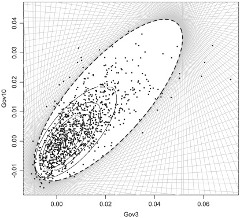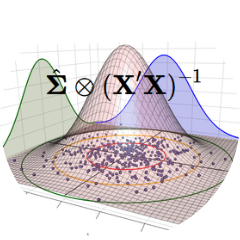Recent Publications

• Double Poisson-Tweedie Regression Models

• Quasi-beta Longitudinal Regression Model Applied to Water Quality Index Data

• Likelihood analysis for a class of Simplex mixed models

• Flexible quasi-beta regression models for continuous bounded data

ProjectsMultivariate marginal models for twin data

The main goal of this project is to propose a new class of multivariate regression models to deal with Gaussian and non-Gaussian data.Construction of multivariate probability distributions.

The main goal of this project is to construct multivariate probability distributions based on the class of exponential dispersion models.Multivariate Covariance Generalized Linear Models

The main goal of this project is to explore the McGLM framework for the analysis of non-trivial data sets and improving the implementation of the mcglm package for the statistical software R.

Teaching

Current courses:

Código Disciplina Curso Turma Ano Semestre
CE224 Métodos Computacional para Inferência Estatística EST 2019 1